# mlptdenoise

Denoise signal using multiscale local 1-D polynomial transform

## Syntax

``y = mlptdenoise(x,t)``
``y = mlptdenoise(x,t,numLevel)``
``y = mlptdenoise(___,Name,Value)``
``````[y,T] = mlptdenoise(___)``````
``````[y,T,thresholdedCoefs] = mlptdenoise(___)``````
``````[y,T,thresholdedCoefs,originalCoefs] = mlptdenoise(___)``````

## Description

example

````y = mlptdenoise(x,t)` returns a denoised version of input signal `x` sampled at the sampling instants, `t`. If `x` or `t` contain `NaN`s, the union of the `NaN`s in `x` and `t` is removed before obtaining the `mlpt`.```

example

````y = mlptdenoise(x,t,numLevel)` denoises `x` down to `numLevel`.```

example

````y = mlptdenoise(___,Name,Value)` specifies `mlpt` properties using one or more `Name,Value` pair arguments, and any of the previous syntaxes```
``````[y,T] = mlptdenoise(___)``` also returns the time instants for the denoised signal.```
``````[y,T,thresholdedCoefs] = mlptdenoise(___)``` also returns the thresholded multiscale local 1–D polynomial transform coefficients.```
``````[y,T,thresholdedCoefs,originalCoefs] = mlptdenoise(___)``` also returns the original multiscale local 1–D polynomial transform coefficients.```

## Examples

collapse all

Denoise a nonuniformly sampled spline signal with added noise using median smoothing and two primal vanishing moments. The nonuniformity of the signal is indicated by NaNs (missing data).

```load nonuniformspline plot(splinenoise) grid on title('Noisy Signal with Missing Data')```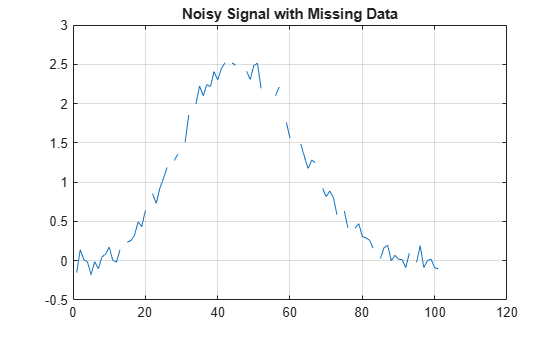Denoise the data using the median denoising method.

`xden = mlptdenoise(splinenoise,[],'DenoisingMethod','median');`

Replace the original missing data in the correct position for plotting purposes. Visualize the original and denoised signals.

```denoisedsig = NaN(size(splinenoise)); denoisedsig(~isnan(splinenoise)) = xden; figure plot([splinesig denoisedsig]) grid on legend('Original Signal','Denoised Signal');```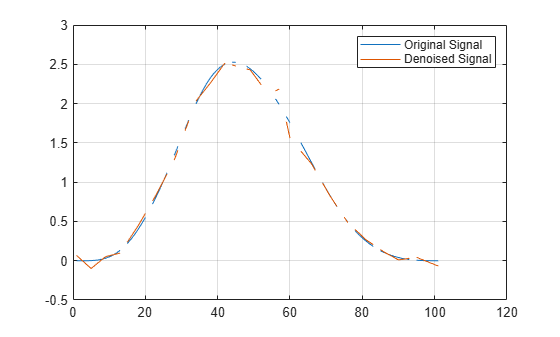Reduce noise of signal using the multiscale local polynomial transform (MLPT).

Load a pure sine wave signal with uniform sampling, and a corrupted version of the signal.

```load('InputSamples.mat') plot(t,x) hold on plot(tCorrupt,xCorrupt) legend('Original','Corrupted')```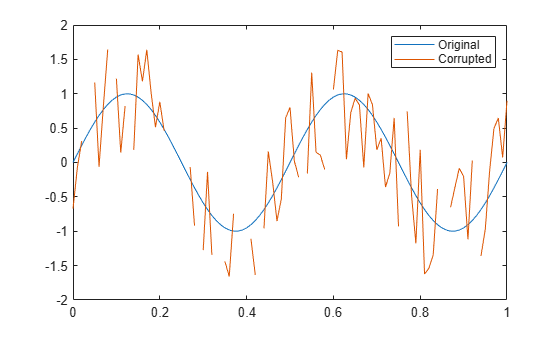Use `mlptdenoise` to denoise the corrupted signal. Visually compare the corrupted and denoised signals against the original signal.

```[xDenoised,tDenoised] = mlptdenoise(xCorrupt,tCorrupt); plot(tDenoised,xDenoised,'b') hold off legend('Original','Corrupted','Denoised')```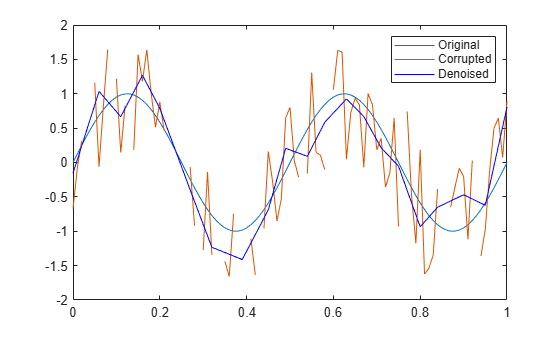Compare the error signals associated with the corrupted signal and the denoised signal. Remove NaNs from the signals for visualization purposes.

```x(samplesToErase) = []; xCorrupt(samplesToErase) = []; xCorruptError = abs(diff([x,xCorrupt],[],2)); yError = abs(diff([x,xDenoised],[],2)); plot(tDenoised,xCorruptError) hold on plot(tDenoised,yError) title('Error Signals') legend('Corrupted','Denoised') hold off```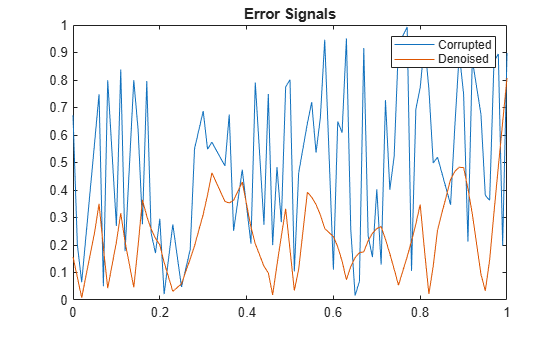By default, `mlptdenoise` denoises a signal based on the two highest-level detail coefficients. In this example, you denoise a signal to different levels and visualize the effect.

Create a multitone signal.

```fs = 1000; t = 0:1/fs:1; x = sin(4*pi*t) + sin(120*pi*t) + sin(480*pi*t);```

Denoise the signal to levels one, two, and five.

```y1 = mlptdenoise(x,t,1); y2 = mlptdenoise(x,t,2); y5 = mlptdenoise(x,t,5);```

Visualize the effect of `level` on the denoised signal.

```subplot(4,1,1) plot(t,x) title('Original Signal') subplot(4,1,2) plot(t,y1) title('Denoised Signal, Level = 1') subplot(4,1,3) plot(t,y2) title('Denoised Signal, Level = 2') subplot(4,1,4) plot(t,y5) title('Denoised Signal, Level = 5')```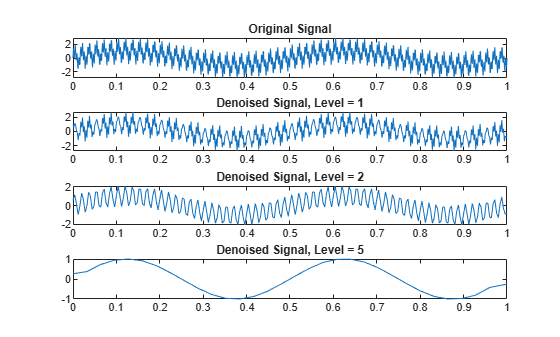The `mlptdenoise` function performs the forward MLPT, thresholds the coefficients as specified by the `'DenoisingMethod'` name-value pair. Then `mlptdenoise` performs the inverse MLPT to return a denoised signal in the domain of your original signal.

You can optionally return the thresholded and original coefficients for inspection and analysis.

Denoise a nonuniformly sampled signal using Stein's unbiased risk method. Return the denoised signal, the associated time instants, the thresholded MLPT coefficients, and the original MLPT coefficients. Plot the original and denoised signals.

```load nonuniformheavisine; [xDenoised,t,wThrolded,wOriginal] = mlptdenoise(x,t,3,'denoisingmethod','SURE'); plot(t,[f,xDenoised]) legend('Original signal','Denoised signal')```Plot the original MLPT coefficients and the thresholded MLPT coefficients for comparison.

```plot([wOriginal,wThrolded]) legend('Original coefficients','Thresholded coefficients')```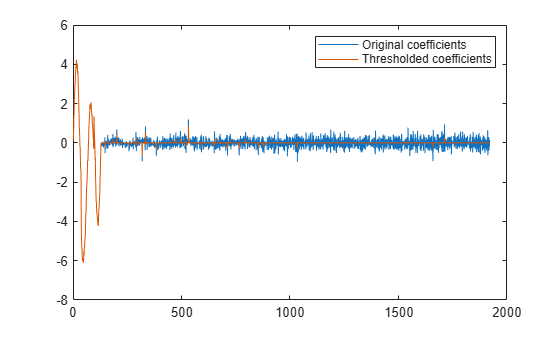## Input Arguments

collapse all

Input signal, specified as a vector or matrix.

• matrix — `x` must have at least two rows. `mlpt` operates independently on each column of `x`. The number of elements in `t` must equal the row dimension of `x`. Any `NaN`s in the columns of `x` must occur in the same rows.

• vector — `x` and `t` must have the same number of elements.

Data Types: `double`

Sampling instants corresponding to the input signal, specified as a vector, `duration` array, or `datetime` array of monotonically increasing real values. The default value depends on the length of the input signal, `x`.

Data Types: `double` | `duration` | `datetime`

Number of resolution levels, specified as a positive integer. The maximum value of `numLevel` depends on the shape of the input signal, `x`:

• matrix — `floor(log2(size(x,1)))`

• vector — `floor(log2(length(x)))`

`mlptdenoise` denoises `x` by thresholding all detail coefficients of an MLPT calculated for `numLevel` resolution levels.

Data Types: `double`

### Name-Value Pair Arguments

Specify optional comma-separated pairs of `Name,Value` arguments. `Name` is the argument name and `Value` is the corresponding value. `Name` must appear inside quotes. You can specify several name and value pair arguments in any order as `Name1,Value1,...,NameN,ValueN`.

Example: `'DualMoments',3` computes a transform using three dual vanishing moments.

Number of dual vanishing moments in the lifting scheme, specified as the comma-separated pair consisting of `'DualMoments'` and `2`, `3` or `4`.

Data Types: `double`

Number of primal vanishing moments in the lifting scheme, specified as the comma-separated pair consisting of `'PrimalMoments'` and `2`, `3`, or `4`.

Data Types: `double`

Prefilter before `mlpt` operation, specified as the comma-separated pair consisting of`'Prefilter'` and `'Haar'` or `'UnbalancedHaar'`. If no prefilter is specified, `'Haar'` is used by default.

Data Types: `char` | `string`

Denoising method applied to MLPT detail coefficients, specified as the comma-separated pair consisting of `'DenoisingMethod'` and `'Bayesian'`, `'Median'`, `'SURE'`, or `'FDR'`.

Note

`'FDR'` has an optional argument for the Q-value. Q is the proportion of false positives and is specified as a real-valued scalar between zero and one. To specify `'FDR'` with a Q-value, use a cell array, where the second element is the Q-value, for example `'DenoisingMethod',{'FDR',0.01}`. If unspecified, Q defaults to `0.05`.

Data Types: `char` | `string`

## Output Arguments

collapse all

Denoised version of the input signal, returned as a vector or matrix. The size of y depends on the size of `x` and the union of `NaN`s in `x` and `t`.

By default, the `mlpt` is denoised based on the two highest resolution detail coefficients, unless `x` has fewer than four samples. If `x` has fewer than four samples, the `mlpt` is denoised based only on the highest resolution detail coefficients.

Data Types: `double`

Sampling instants corresponding to the output, returned as a vector or `duration` array obtained from `x` and the input `t`. If the input `t` is a `datetime` or `duration` array, `t` is converted to units that enable stable `mlpt` and `implt` computation. Then `T` is returned as a `duration` array.

Data Types: `double` | `duration`

Thresholded MLPT coefficients, returned as a vector or matrix. The size of `thresholdedCoefs` depends on the size of `x` and the level to which the transform is calculated.

Data Types: `double`

Original MLPT coefficients, returned as a vector or matrix. The size of `originalCoefs` depends on the size of `x` and the level to which the transform is calculated.

Data Types: `double`

## Algorithms

Maarten Jansen developed the theoretical foundation of the multiscale local polynomial transform (MLPT) and algorithms for its efficient computation . The MLPT uses a lifting scheme, wherein a kernel function smooths fine-scale coefficients with a given bandwidth to obtain the coarser resolution coefficients. The `mlpt` function uses only local polynomial interpolation, but the technique developed by Jansen is more general and admits many other kernel types with adjustable bandwidths .

 Jansen, M. "Multiscale Local Polynomial Smoothing in a Lifted Pyramid for Non-Equispaced Data". IEEE Transactions on Signal Processing, Vol. 61, Number 3, 2013, pp. 545–555.

 Jansen, M., and M. Amghar. "Multiscale local polynomial decompositions using bandwidths as scales". Statistics and Computing (forthcoming). 2016.

 Jansen, M., and Patrick Oonincx. Second Generation Wavelets and Applications. London: Springer, 2005.

## SupportGet trial now